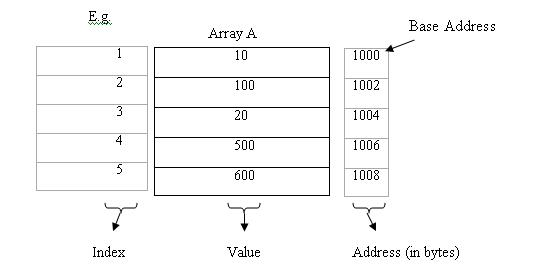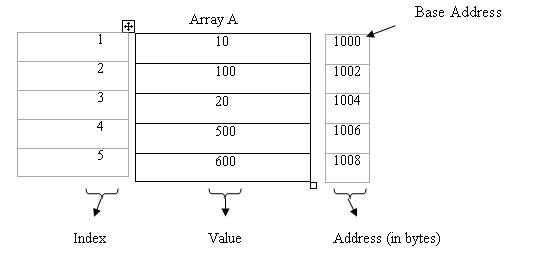(a) Subscript or Index: It is the sequence number of an element in array. Each element of array is represented by a subscripted variable which is formed as: A [K] Here A is array name. K is subscript or index. A [K] is the subscripted variable. E.g. The 10 elements of array A will be represented by their corresponding subscripted variables as: A , A , A , ….. , A . A  has value of first element. A  has value of second element. And so on. (b) Size or Length of array : It means the number of elements in the array. It can be calculated by using the following formula: Length or Size = UB – LB + 1 Here UB is upper bound and is the largest or uppermost index of array. LB is lower bound and is the smallest or lowest index of array. E.g. We have an array A . In it the elements are from A  to A . So its LB is 1 and UB is 10. The size of this array will be: Size = 10 - 1 + 1 = 10 elements (c) Base Address of array: Each element of array has an address. Base address of array is the address of first element of the array. On the basis of it we can find the address of any element.Here 1000 is the base address of A because it is the address of first element of A. (Note: An integer takes 2 bytes in the memory. So in above example the difference between addresses is of 2.) (d) Offset: Offset of an element is the distance or difference between the base address of array and the address of the element. By using the base address and offset, we can find the address of any element of the array.The address of 4th element is 1006 and the base address of A is 1000. So the offset for 4th element is 1006 – 1000 = 6 bytes. Share this article   |    Print    |    Article read by 4014 times Author:Rohit kakria I am software developer Related Articles: No related article Related Interview Questions: No related interview question